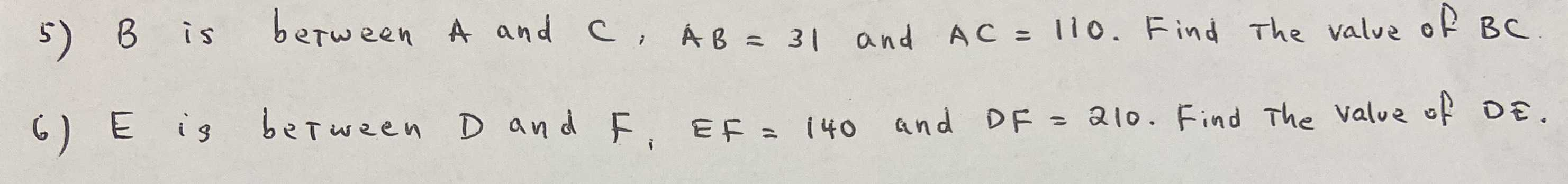### ¿Todavía tienes preguntas de matemáticas?

Pregunte a nuestros tutores expertos
Algebra
Pregunta5) $$B$$ is between $$A$$ and $$C , A B = 31$$ and $$A C = 110$$ . Find the value of $$B C$$ . 6) $$E$$ is between $$D$$ and $$F , E F = 140$$ and $$D F = 210$$ . Find the value of $$D E$$ .

5) $$BC= AC- AB= 79$$
6) $$DE= DF- EF= 70$$